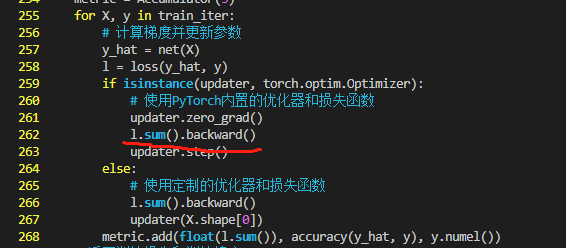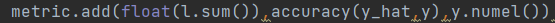# Softmax回归的从零实现

TypeError: float() argument must be a string or a number, not ‘builtin_function_or_method’1 Like

@CHENHUI_XU 这节里的loss不是torch里的loss，是自己定义的函数，求出来的就是张量，如果用的torch里的loss默认才是均值。所以这里使用torch的sgd。需要l.mean()
@Ye_Zhang 转换成什么形式（sum or mean）要看sgd是如何定义的吧，如果用torch的sgd就是mean，d2l里的sgd就是sum？
@Yuchen971 你更新一下d2l的代码，“0.17.1的d2l包封装的pytorch的训练模型迭代的代码有一个小问题，train_epoch_ch3函数的第9行代码应该是“l.mean().backward()，d2l包里是l.sum()”``````import torch
def crossEntropyNaive(x):
expX = torch.exp(x)
return expX / expX.sum(axis = 1, keepdim=True)

def crossEntropySlightlySmart(x):
row_max,_ = torch.max(X, dim = 1, keepdim=True)
x = x - row_max
expX = torch.exp(x)
return expX / expX.sum(axis = 1, keepdim=True)

X = torch.tensor([[1.0,1.0],[1.0,10.0],[1.0,100.0]])
print(crossEntropyNaive(X))
print(crossEntropySlightlySmart(X))
``````

d2l包里的torch.py源代码的show_images()函数里，在最后一行前加plt.show()